## Introduction

Gate-defined semiconductor quantum dots enable the confinement and manipulation of individual electrons and their spin1. Most of the relevant parameters – electron filling, energy splittings, spin states, exchange interaction – can be tuned in situ by electric and magnetic fields. Because of this exquisite level of control, quantum dots are being investigated as candidate systems for spin-based quantum information processing2. In group III-V semiconductors such as GaAs, the development of highly tunable double quantum dots has allowed the study of both single-electron and two-electron spin dynamics3,4,5,6,7. However, the nuclear spins always present in these materials produce strong decoherence of the electron spin degree of freedom and result in phase coherence times T2 of below 1 ms8,9. Conversely, group-IV semiconductors such as silicon, silicon-germanium and carbon can be isotopically purified, leaving only spinless isotopes. The weak spin-orbit coupling10 and the absence of piezoelectric electron-phonon coupling11 allow for extremely long spin relaxation times T1 of order seconds, as already demonstrated in several experiments12,13,14. The phase coherence times have not been measured yet, but they are expected to reach 1 s as well, in highly purified 28Si substrates with low background doping concentration15.

A widely successful method to observe and control spin phenomena in quantum dots1 consists of defining a double quantum dot in a series configuration and tuning the potentials such that sequential electron transport requires a stage where two electrons must occupy the same dot. The eigenstates of a two-electron system are singlet and triplet spin states, separated by an energy splitting ΔST which can be large in tightly confined dots. The electron transport then becomes spin-dependent and can be blocked altogether when the two-electron system forms a triplet state5,16. This phenomenon, known as Pauli spin blockade, has been extensively exploited to investigate the coherence of single-spin4 and two-spin states3 in GaAs and InAs17 quantum dots. Therefore, observing and controlling spin blockade in silicon is a key milestone to unravel the full potential of highly coherent spin qubits. Preliminary success has been obtained in Si18 and SiGe19 devices, but in each case the double dot system under study resulted from local variations in the potential of a lithographically-defined single dot, making it difficult to control individual dot occupancies or inter-dot coupling. Spin-based quantum dot qubits require exquisite control of these parameters, so a highly tunable double-dot system in silicon is essential. For singlet-triplet qubits in multivalley semiconductors it is also crucial to ensure that a large valley-orbit splitting is present, to avoid the lifting of Pauli blockade due to valley degeneracy20,21.

Here we present an engineered silicon double quantum dot which shows excellent tunability and robust charge stability over a wide range of electron occupancy (m, n). The silicon metal-oxide-semiconductor (MOS) structure utilizes an Al-Al2O3-Al multi-gate stack that enables very small dots to be defined, each with independent gate control, together with gate-tunable inter-dot coupling. Such multi-gate stacks have previously been used to construct single Si quantum dots with the ability to achieve single electron occupancy22. The double dot presented here exhibits spin blockade in the few-electron regime, from which we are able to extract a large singlet–triplet energy splitting and also investigate a new mechanism giving rise to singlet–triplet transitions in the weak-coupling regime.

## Results

### Device architecture

Figure 1 shows a scanning electron micrograph (SEM) and cross-sectional schematic of the device, which incorporates 7 independently controlled aluminium gates. When a positive bias is applied to the lead gates (L1 and L2) an accumulation layer of electrons is induced under the thin SiO2, to form the source and drain reservoirs for the double dot system. A positive voltage on the plunger gate P1 (P2) causes electrons to accumulate in Dot 1 (Dot 2). Independent biasing of P1 and P2 provides direct control of the double-dot electron occupancy (m, n). The tunnel barriers between the two dots and the reservoirs are controlled using the barrier gates: B1, B2 and B3. The middle barrier gate B2 determines the inter-dot tunnel coupling. The electrochemical potentials of the coupled dots can also be easily tuned to be in resonance with those of the source and drain reservoirs. As shown in Fig. 1(b), gates L1 and L2 extend over the source and drain n+ contacts and also overlap gates B1 and B3. The upper-layer gates (P1 and P2) are patterned on top of the lead and barrier gates. The lithographic size of the dots is defined by the distance between adjacent barrier gates (30 nm) and the width of the plunger gates (50 nm), as shown in Fig. 1(a).

### Inter-dot tunnel coupling tunability

Figure 2 shows the measured differential conductance of the device as a function of the plunger gate voltages, VP1 and VP2, with all other gate voltages held constant, together with sketches of the energy landscape of the double dot. The charge-stability maps moving from Fig. 2(a) to 2(c) clearly show the effects of an increasing inter-dot coupling as the middle barrier-gate voltage VB2 is increased, lowering the tunnel barrier between the dots. Fig. 2(b) shows the characteristic honeycomb-shaped stability map representing intermediate inter-dot coupling23, obtained at VB2 = 1.32 V. At lower middle barrier-gate voltage, VB2 = 1.20 V, we observe a checker-box shaped map [Fig. 2(a)], since the middle barrier is opaque enough to almost completely decouple the two dots. In contrast, the stability map in Fig. 2(c) shows the formation of diagonal parallel lines at VB2 = 1.40 V. Here the two dots effectively merge into a single dot due to the lowering of the middle barrier [Fig. 2(f)]. Increasing VB2 further results in stronger differential-conductance lines, suggesting a simultaneous increase in dot-lead couplings. The transport measurements shown here do not allow a precise determination of the electron occupancy (m, n) in the dots, since it is possible that electrons remain in the dots even when ISD is immeasurably small. For the regime plotted in Fig. 2 there were at least 10 electrons in each dot, based on our measurement of Coulomb peaks as we further depleted the system. An absolute measurement of dot occupancy would require integration of a charge sensor into the system7. These results nevertheless demonstrate that the multi-gated structure provides excellent tunability of coupling while maintaining charge stability over a wide range of electron occupancy.

### Capacitances and charging energies

Application of a DC source-drain bias VSD causes the triple-points in the weakly-coupled regime [Fig. 2(a)] to extend to form triangular shaped conducting regions [Fig. 3(a)] from which the energy scales of the double dot system can be determined23. From a triangle pair, we extract the conversion factors between the gate voltages and energy to be α 1 = eVSD/δVP1 = 0.089e and α 2 = eVSD/δVP2 = 0.132e, where δVP1 and δVP2 are the lengths of the triangle edges, as shown in Fig. 3(a). The total capacitances of Dot-1 and Dot-2 can then be calculated23, giving C1 = 16.3 aF and C2 = 14.5 aF. The accuracy of these values is around 5%, limited by the accuracy with which our data can be fitted by the superimposed (dotted) triangles in Fig. 3(a).

From the above analysis, we find the charging energies of the two dots to be EC,1 = e2/C1 = 9.8 meV and EC,2 = e2/C2 = 11 meV, indicating that the left dot is slightly larger than the right dot. We note that a previous study for a single quantum dot22 reported a charging energy of 6 meV at an electron occupancy of 40 electrons. In Fig. 3 we estimate an electron occupancy of 10 or less in each dot and the larger charging energies measured here are consistent with the lower occupancy and hence smaller size, of these dots.

Figure 3 shows the current ISD through the double dot as a function of the two plunger gate voltages when measured with both positive [Fig. 3(a)] and negative [Fig. 3(b)] source-drain biases. Here we observe a suppression of current at one bias polarity, the characteristic signature of Pauli spin blockade6,7. At VSD = +2.5 mV we observe a pair of overlapping full bias triangles, as shown in Fig. 3(a). Resonant transport through the ground state and the excited states in the double dot occurs when the states within the dots are exactly aligned, leading to peaks in the current which appear as straight lines parallel to the triangle base in Fig. 3(a). The non-resonant background current level at the centre of the triangle is attributed to inelastic tunneling. The non-zero current throughout the triangular region indicates that electrons from the reservoir can tunnel freely from the S(0,2) singlet state to the S(1,1) singlet state, as depicted in the cartoon (red box in Fig. 3). Note that here we define (m, n) as the effective electron occupancy18, while the true electron occupancy is (m+m0, n+n0). The Pauli blockade expected for two-electron singlet and triplet states occurs when the total electron spin of each dot is zero in the (m0, n0) state.

At the complementary negative bias of VSD = −2.5 mV we observe strong current suppression in the region bounded by the dashed lines in Fig. 3(b). The suppression arises because the transition from T(1,1) to S(0,2) is forbidden by spin conservation during electron tunneling. Once the T(1,1) triplet state is occupied, further current flow is blocked until the electron spin on one dot reverses its orientation via a relaxation process (green star box in Fig. 3)6,7.

Note that for both positive VSD [Fig. 3(a)] and negative VSD [Fig. 3(b)] the current ISD increases as VP1 and VP2 increase, leading to apparent asymmetry in the bias triangles, with the highest currents in the top-right of both figures. For VSD = −2.5 mV this leads to a weak conducting region (light red) at the base of the bias triangle. The asymmetry indicates that the double dot system is more strongly coupled to the drain contact than to the source.

### Singlet-triplet splitting

In a magnetic field B there are four accessible spin states: the singlet S; and three triplets T, T0 and T+, corresponding to SZ = −1, 0, +1. The singlet–triplet splitting ΔST is the energy difference between the blockaded ground state S(0,2) and the excited state T(0,2)7,18. Here we study ΔST as a function of B, applied parallel to the substrate, by measuring spin blockade at a negative bias. Figures 4(a–c) show the bias triangles in the spin blockade regime at increasing magnetic fields B = 2, 4 and 6 T, with the splitting ΔST marked in Fig. 4(a). For |B| > 700 mT the current in the spin-blockaded region is fully suppressed and so, in order to identify the base of the effective bias triangles [dotted lines in Figs. 4(a–c)], we use the dimensions of the (non-blockaded) bias triangles for VSD = +2.5 mV and align these to the visible peaks of the triangles for VSD = −2.5 mV. The measured splitting ΔST decreases linearly with increasing B [Fig. 4(d)], as expected, since the triplet states split linearly by the Zeeman energy, EZ = ±SZ|gBB, where µB is the Bohr magneton and SZ is −1, 0, +1. A linear fit through ΔST(B) yields a Landé g-factor of 2.1 ± 0.2, consistent with electrons in silicon.

We observe a very large value of the (0,2) singlet-triplet splitting at B = 0, ΔST ≈ 1.4 meV. If this were a true two-electron double quantum dot, the result would imply that the nearest valley-orbit state was at least 1.4 meV above the ground state. The first excited valley-orbit state should be a combination of the ±z valleys and would lift the spin blockade20,21, showing no remarkable energy shift in a magnetic field, however, since the electron occupancy in our dots is unknown and larger than two, no conclusive statements can be made on the absence of low-lying valley-orbit states.

### Leakage current in blockade regime

If some mechanism exists to mix the singlet and triplet states or to induce transitions between them, then the spin blockade can be lifted, leading to a measurable leakage current6. Here, we observe leakage currents in the spin blockade regions for low values of magnetic field, |B| < 700 mT. Fig. 5(a) shows the surface plot of the leakage current ISD as a function of both detuningand magnetic field B, while Figs. 5(b) and 5(c) show line traces of ISD as a function of B at zero detuning and ISD as a function ofat zero magnetic field, respectively. We find that the leakage current has a maximum at B ≈ 0 and falls to zero at |B| 700 mT. As discussed below, we find that the transition from triplet to singlet is well explained by spin-flip cotunneling24, resulting in a non-zero time-averaged leakage current via the mechanism illustrated in Figure 5(d).

## Discussion

The suppression of leakage current by an applied magnetic field has been observed in GaAs double quantum dots6 and attributed to the effect of hyperfine coupling between the electron spins and the surrounding bath of nuclear spins. In that case the width δB of ISD(B) yields the average strength of the hyperfine field. For an unpolarized nuclear spin bath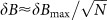, where δBmax is the hyperfine field assuming fully polarized nuclei and N is the number of nuclei overlapping with the electron wave function. For a typical GaAs dot overlapping with 106–107 nuclei, δBmax 6 T δB 2–6 mT6,7,25. In natural silicon, however, the hyperfine interaction is much smaller than in GaAs, with δBmax ≈ 1.9 mT26. Here instead we found that B > 300 mT is necessary to suppress the leakage current. Therefore, hyperfine coupling can be ruled out as a mechanism for the lifting of spin blockade.

An alternative mechanism for a transition from triplet to singlet has been recently proposed, where the spin flip is caused by inelastic cotunneling involving one of the leads24. The spin-flip rates due to cotunneling from the spin-polarized triplet states, T±(1,1), are exponentially suppressed when the Zeeman energy is large compared to the thermal broadening of the electron states in the leads (i.e., for BB > kBT, where T is the electron temperature and B is the applied magnetic field). This is because the excitation processes on the dot require the removal of an electron from above the lead Fermi level and the creation of an electron below the Fermi level [see diagram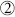in Fig. 5(d)].

A rate-equation analysis accounting for the energy dependence of the spin-flip cotunneling rates24 then gives a simple form in the limit of weak inter-dot tunneling t and weak cotunneling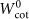compared to the tunnel rates ΓS,D between a dot and its nearby source or drain lead (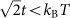,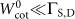):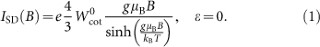Here, the B = 0 spin-flip cotunneling rate (for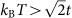and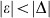, |eVSD|) is: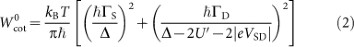with mutual (inter-dot) charging energy U′ and Δ = α 1δVP1 + α 2δVP2 for plunger gate voltages δVP1,P2 measured from the effective (0, 1) – (1, 1) – (0, 2) triple point (lower-left corner of the bias triangle in Fig. 3(b)). Eq. (2) accounts for virtual transitions between effective (1, 1) and (0, 1) (first term) as well as effective (1, 1) and (1, 2) charge states (second term).

In the present case, Δ |eVSD| U′. The higher current level in the upper right corner of Fig. 3(b) further suggests ΓD ΓS, giving (for this particular experiment):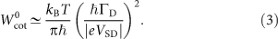Using the above expression for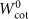, we then use Eq. (1) to fit to the ISD(B) data in Fig. 5(b), giving us ΓD = 30 µeV for the tunneling rate and T = 155 mK for the electron temperature.

The B = 0 spin-flip cotunneling rateis energy-independent in the limit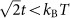. However, the leakage current does acquire a dependence on the energy detuning,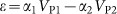, when the escape rate from the double-dot due to resonant tunneling is suppressed below the spin-flip cotunneling rate. This leads to a Lorentzian dependence of the current on detuningwith a t-dependent width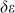: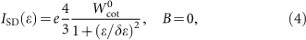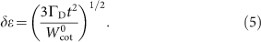Eq. (4) is valid in the same limit (,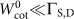) as Eq. (1). In the strong-tunneling limit,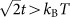, the theory predicts that I() should show a strong resonant-tunneling peak of width t, followed by a slowly-varying Lorentzian background described by Eq. (4) at large. The absence of a strong resonant-tunneling peak in the data of Fig. 5(c) confirms that the device is operating in the regime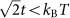, justifying our use of Eqs. (1) and (4) to analyse the data.

A nonlinear fit to the ISD() data [for VB2 = 1.1176 V in Fig. 5(c)] using Eq. (4) yields t = 0.5 µeV for the inter-dot tunneling rate, using our previously determined values ΓD = 30 µeV and T = 155 mK. These parameter values are well within the experimentally expected range. The small value of t indicates weak inter-dot tunnel coupling, consistent with the results shown in Fig. 3(b). By independently tuning the inter-dot tunnel coupling via control of the middle barrier gate voltage, we have verified that by increasing VB2 to 1.1186 V, the leakage current acquires a higher absolute value and a broader B-dependence [blue trace in Fig. 5(c)], as predicted. We note that as VB2 is made more positive, the interdot coupling t increases as expected and the dot-lead couplings ΓD also increase somewhat. We conclude that the spin-flip cotunneling mechanism provides a consistent explanation of the observed leakage current in the spin blockade regime. The mechanism could be applied to reanalyse previous experiments in group IV semiconductors27 where the nature of the leakage current was not fully understood.

In conclusion, we have presented a lithographically-defined double quantum dot in intrinsic silicon showing excellent charge stability and low disorder. The multi-gate architecture provides independent control of electron number in each dot as well as a tunable tunnel coupling. We observed Pauli spin blockade in an effective two-electron system from which we extracted the singlet–triplet splitting. The leakage current in the spin blockade regime is well explained by a spin-flip cotunneling mechanism, which could be of widespread importance in group-IV materials with weak hyperfine coupling. The results obtained here provide a pathway towards investigation of spin blockade in silicon double quantum dots with true (1,1) and (2,0) electron states. Towards this end, we are planning future experiments incorporating a charge sensor to monitor the last few electrons28. We anticipate that such an architecture will provide excellent prospects for realising singlet–triplet qubits in silicon29.

## Methods

### Fabrication steps

The devices investigated in this work were fabricated on a 10 kΩ-cm n–type high resistivity 〈100〉 silicon wafer using standard micro-fabrication techniques. The n+ source and drain ohmic contacts regions in Fig. 1(b) were produced via high concentration phosphorus diffusion at 1000°C, resulting in peak dopant densities of 1020 cm−3. Next, the high-quality SiO2 of 10 nm thickness was grown via dry thermal oxidation in the central region at 800°C in O2 and dichloroethylene. The barrier gates were first patterned on the thin SiO2 region using electron beam lithography (EBL) followed by thermal evaporation of 40 nm thick aluminium and lift-off process. Before the next EBL step, the barrier gates are exposed to air for 10 mins at 150°C to form 4 nm of Al2O3 acting as a dielectric layer. This process was repeated for lead gates and plunger gates layers with aluminium thicknesses of 40 nm and 120 nm respectively. A final forming gas anneal (95% N2 and 5% H2) was performed for 15 mins to achieve a low density of Si-SiO2 interface traps, of order 1010 cm−2 eV−1, as measured on a similarly processed chip30. The low trap density is clearly reflected in the device stability and the low level of disorder observed in the transport data shown in the results section.

### Experimental setup

Electrical transport measurements were carried out in a dilution refrigerator with a base temperature T 100 mK. We simultaneously measured both the DC current and the differential conductance dI/dVsd, the latter using a source-drain AC excitation voltage of 100 µV at 87 Hz.